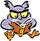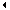HOME / FEEDBACK / ORDER THE BOOK / OTHER BOOKS / THANKS

Day 5: Creating Classes and Methods

Certification Practice

Each chapter of the book ends with a Certification Practice question that's comparable to what you could expect to be asked on a Java certification test. Here's the answer for chapter 5:

Answer (c) is correct. The constructor method of the BiggerValue class calls the method calculateResult(int, int, int). Because this method is not defined in the BigValue class, it must be defined in the BiggerValue class.

Answers (a) is incorrect. It would produce a result equal to 312.0 if the constructor method of the BiggerValue class called calculateResult(int) with the c variable as an argument, but the constructor calls calculateResult(int, int, int).

Answers (a) and (d) are incorrect. Using either of these statements, the example will not compile because the constructor method of the BiggerValue class uses a variable called c which is not defined in the constructor.